Next: 4.3.2 Hopkins' Method Up: 4.3 Advanced Illumination Aperture Previous: 4.3 Advanced Illumination Aperture

## 4.3.1 Abbe's Method

Following Abbe the image due to one coherent source point is first calculated. Either the scalar formulation of (4.54) or the vector form of (4.68) can be used. The aerial image is then obtained by an incoherent superposition of all the contributions and formally writes to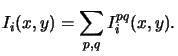(4.73)

The point sources (p, q) are located within the illumination aperture. Beside this no further restrictions on the shape of the aperture are made. Examples for annular and quadrupole illumination are shown in Figure 4.7.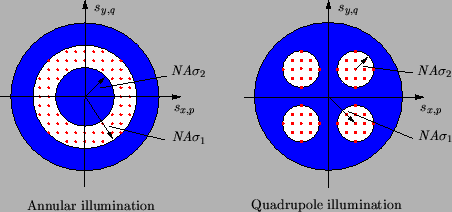The spacing between the individual point sources has to satisfy a specific criterion. As mentioned before, it is chosen in such a way that the lateral wavevector components sx, p and sy, q of the waves incident on the photomask equal an integer multiple of the sampling frequencies M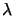/a and M/b (cf. (4.47)). This choice guarantees that the image on the wafer surface is periodic with periods a and b, which becomes extremely important in context with the exposure/bleaching simulation described in Chapter 6. This requirement is illustrated in the wavevector diagram of Figure 4.8 for the case of conventional illumination.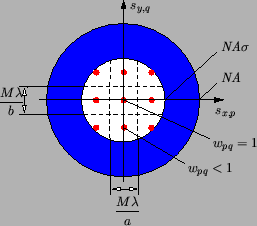What is missing now is the determination of the amplitudes Apq of the point sources. Clearly, they depend on the total imaging intensity I0 as well as on the discretization scheme illustrated in Figure 4.8. The intensity of one point source is proportional to the discretization area wpq. As it is also related to the square of the light amplitude Apq2 we write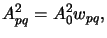(4.74)

where A0 is a simple normalizing constant. By considering a missing or perfectly transparent'' mask, we obtain the relationship between the amplitude Apq and the total imaging intensity I0 looked for.

The transmission characteristic t(x, y) of a transparent mask equals to unity, and its Fourier spectrum Tnm thus consists of a delta impulse at zero frequency, i.e.,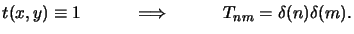(4.75)

The scalar and vector approach have to be studied separately from now on:
• Scalar theory. With (4.84) and (4.85) the image spectrum (4.52) writes as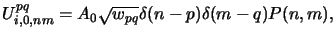(4.76)

and the image amplitude and intensity are obtained from (4.51) and (4.54)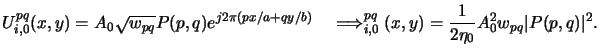(4.77)

The aerial image intensity Ii, 0(x, y) is thus constant and with the definition of the scalar pupil function (4.69) found to be(4.78)

whereby we assume that all point sources (p, q) are collected by the projection lens, i.e., the numerical aperture of the condenser lens must be smaller than that of the projection lens or, equivalently, the partial coherence factordefined in (2.4) is smaller than unity,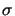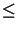1. From that the normalizing constant A0 can simply be determined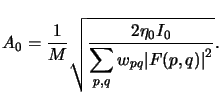(4.79)

• Vector theory. The electric field amplitude (4.57) writes with (4.84) and (4.85) to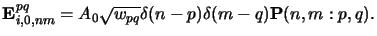(4.80)

Hence, in case of blank illumination a single homogeneous plane wave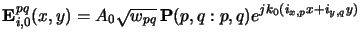(4.81)

is incident on the wafer. For a homogeneous plane wave the vertical component of the Poynting vector is simply given by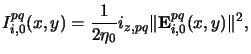(4.82)

and with (4.65) to (4.69) the contribution of a single point source is calculated as follows:n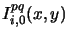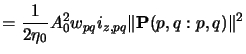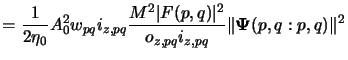(4.83)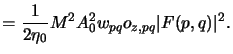As expected the contributions Ipqi, 0(x, y) are constant. Thus we obtain for the blank aerial image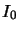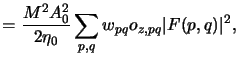(4.84) from which the constant tex2html_wrap_inline$A_0$ can easily be determined,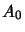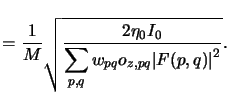(4.85)

#### Footnotes

... follows:n
For (n, m) = (p, q) and thus spq = opq we get from (4.66) and (4.67) |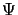(p, q : p, q)|2 = oz, pq2.Next: 4.3.2 Hopkins' Method Up: 4.3 Advanced Illumination Aperture Previous: 4.3 Advanced Illumination Aperture
Heinrich Kirchauer, Institute for Microelectronics, TU Vienna
1998-04-17